# 高级计算机图形学:光线追踪和辐射着色

## Ray Tracing

Posted by R1NG on November 18, 2022 Viewed Times

# 光线追踪与辐射着色

## 光线追踪 Raytracing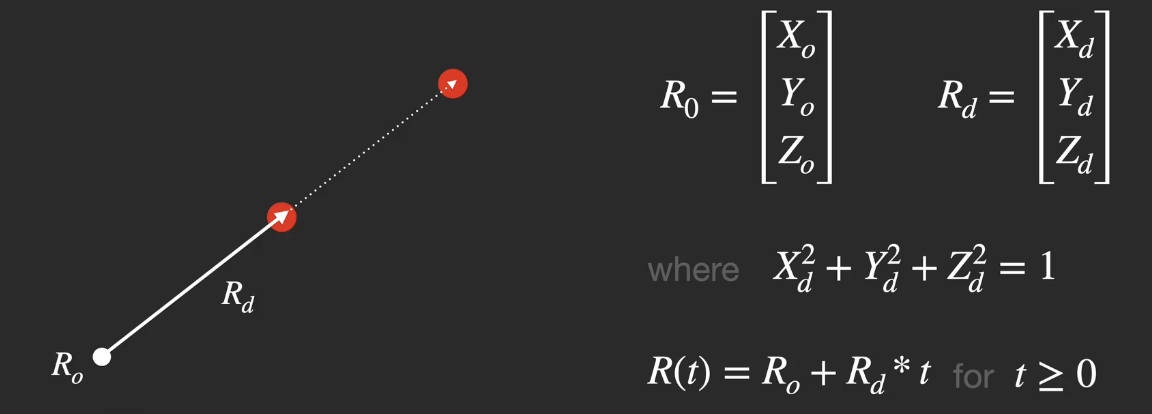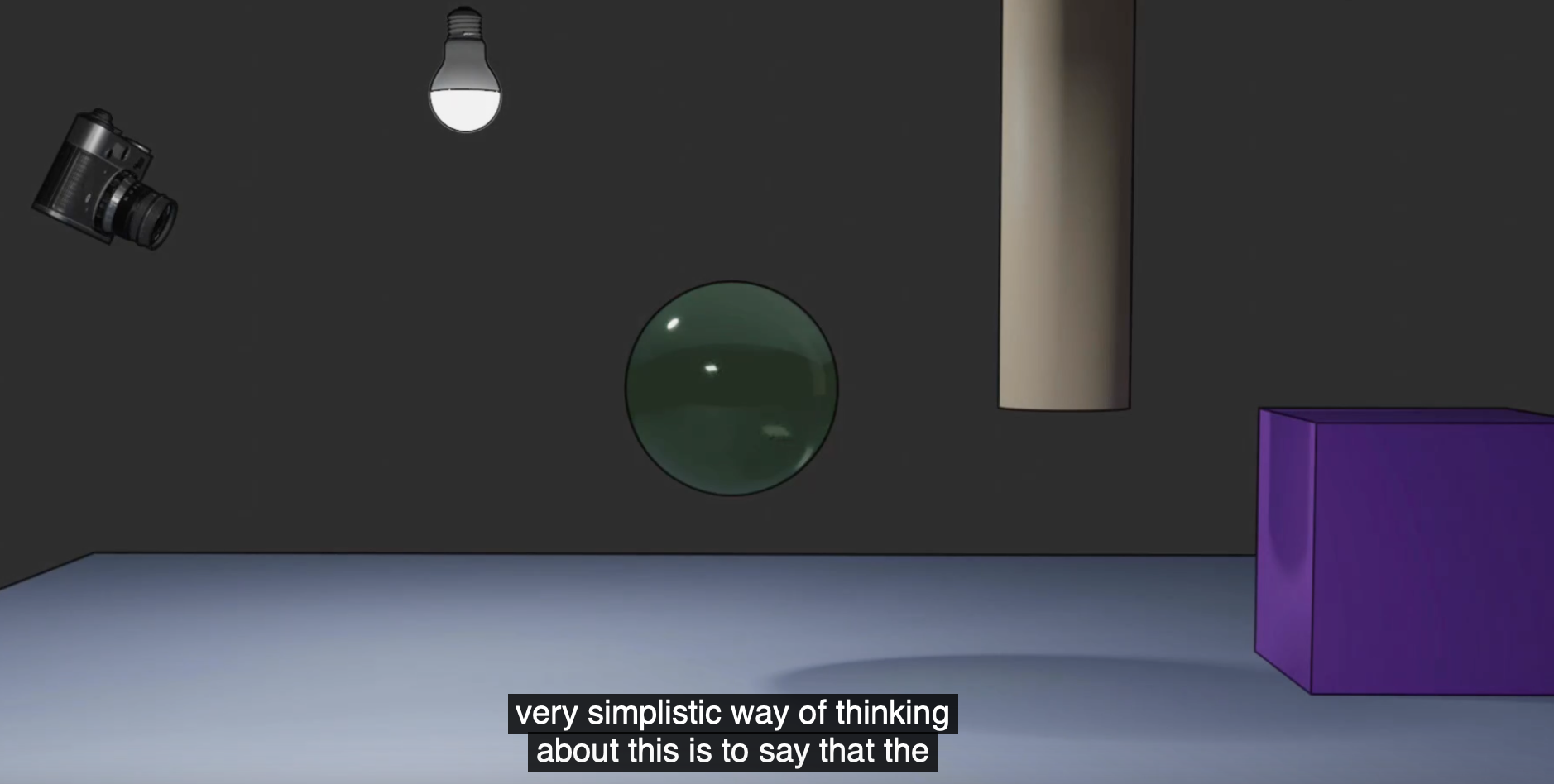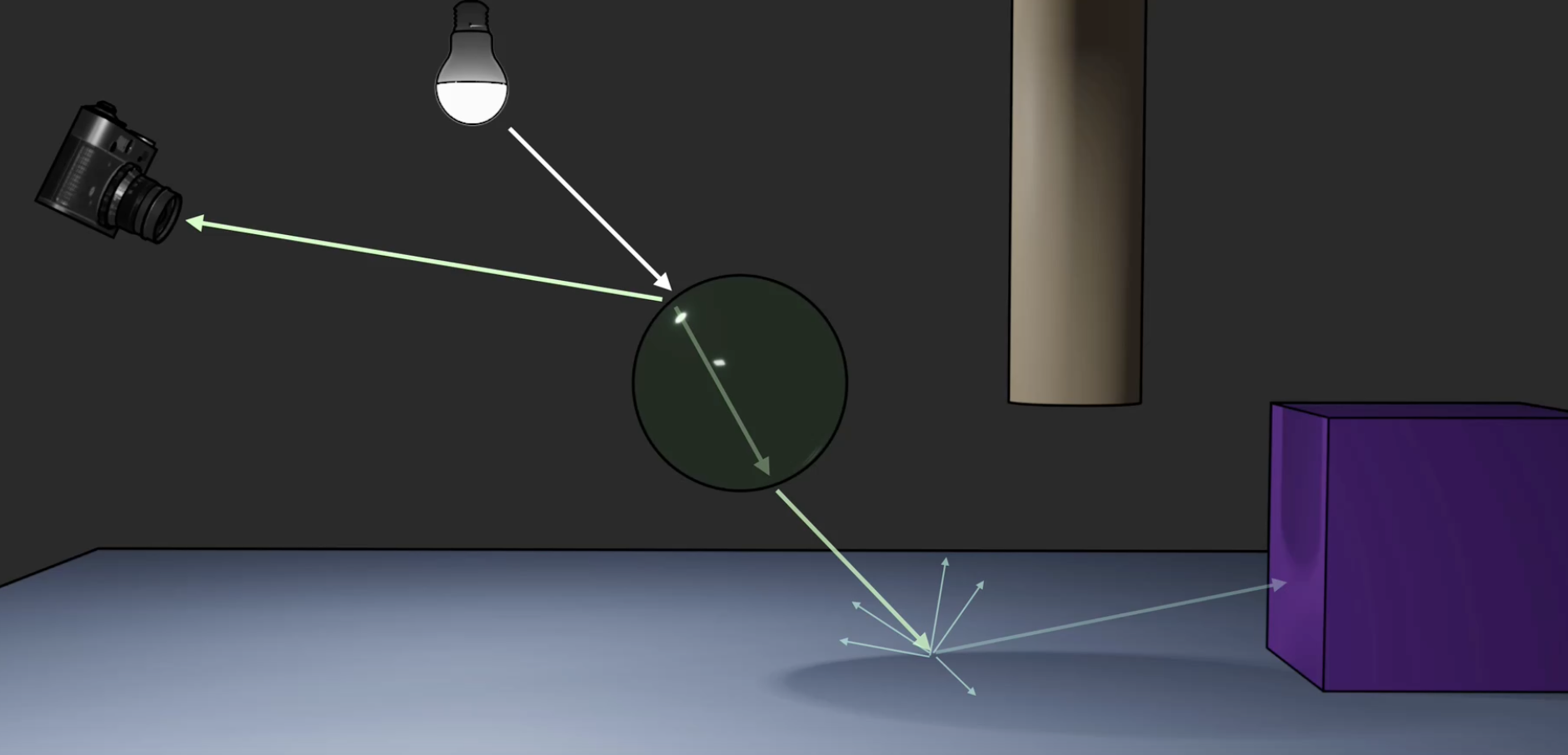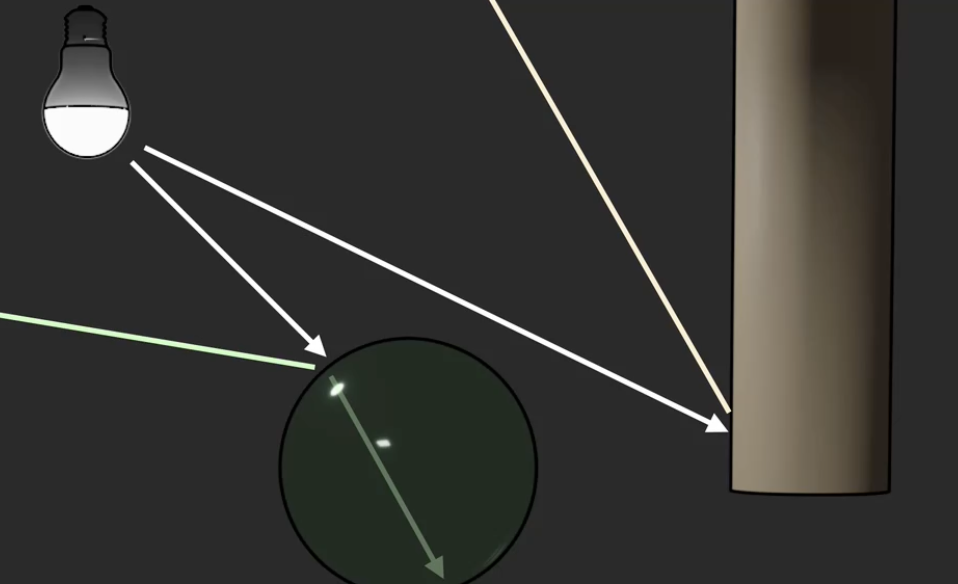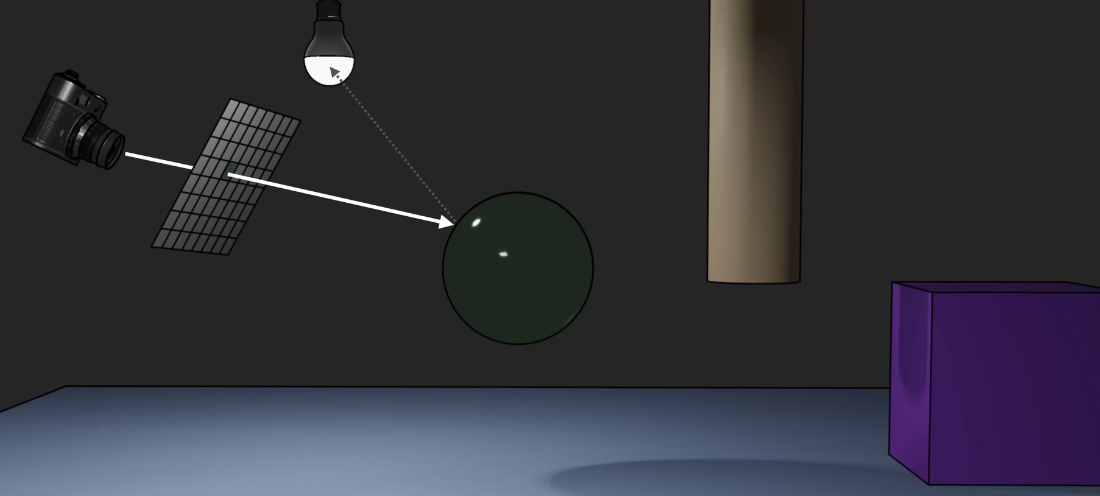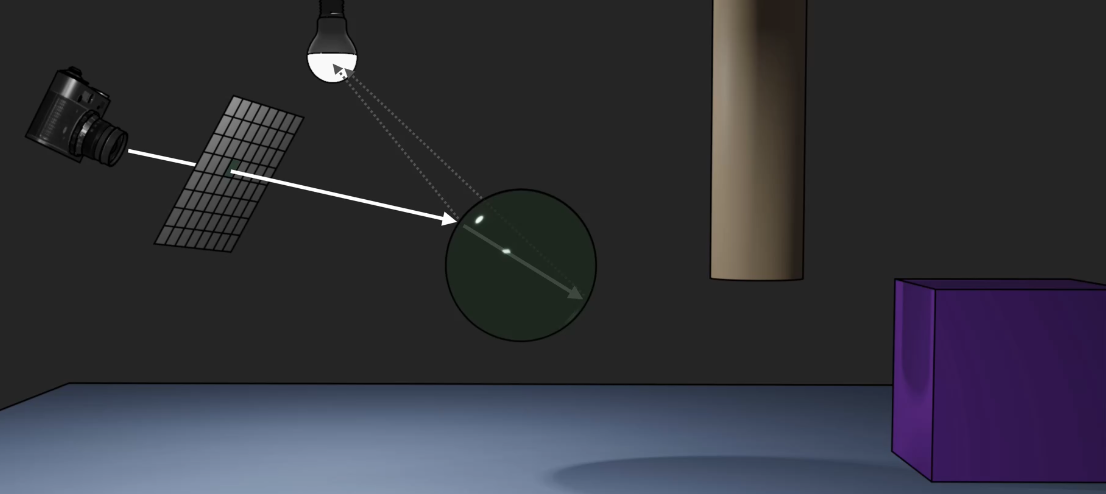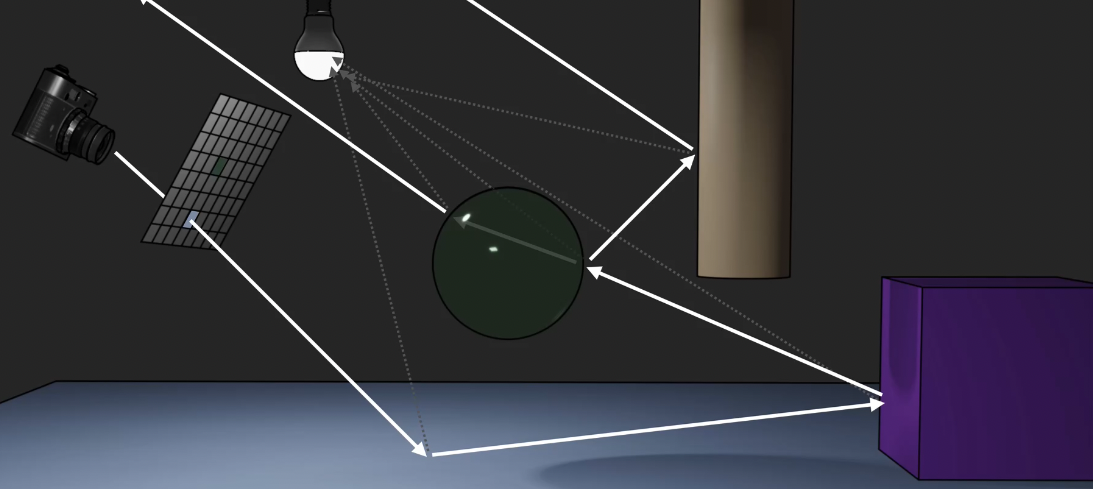## 辐射着色 Radiosity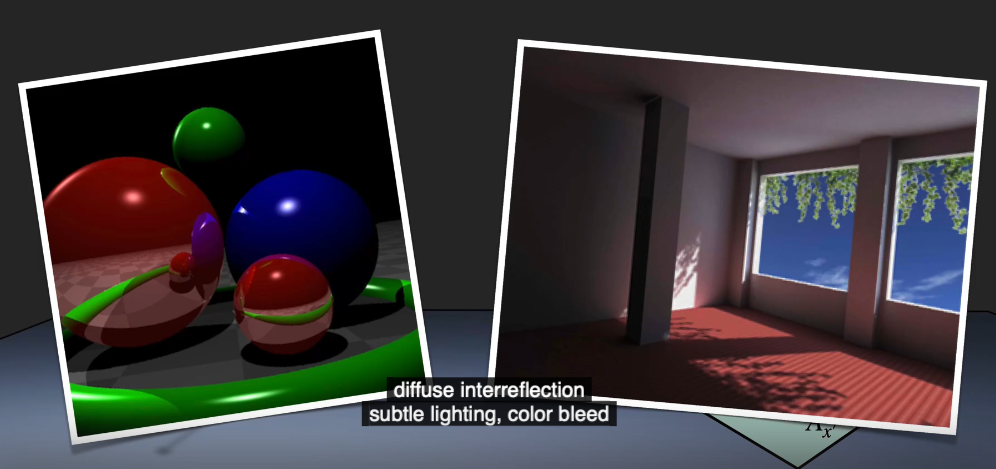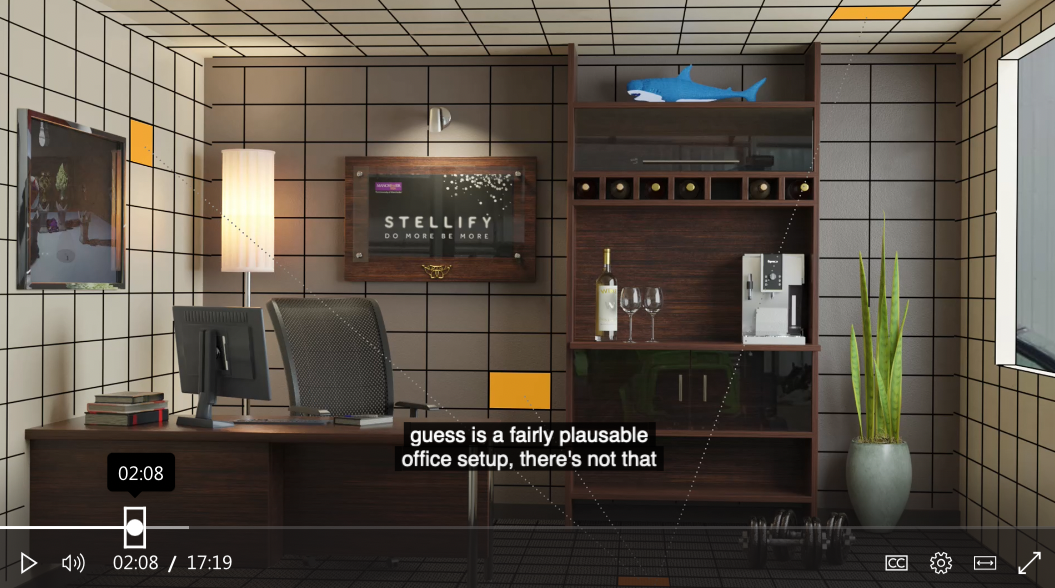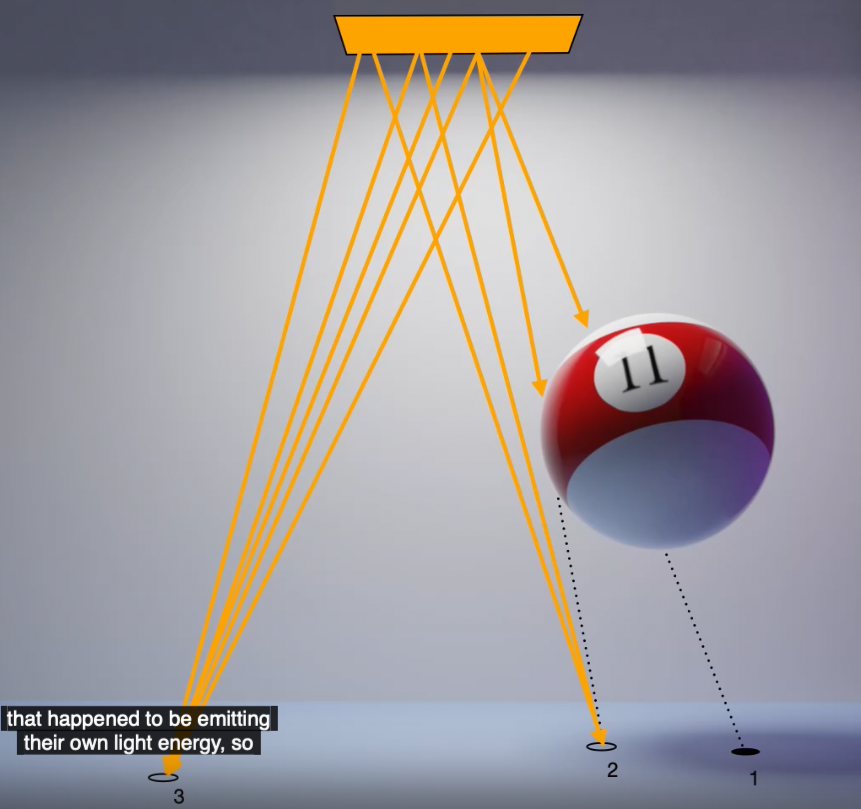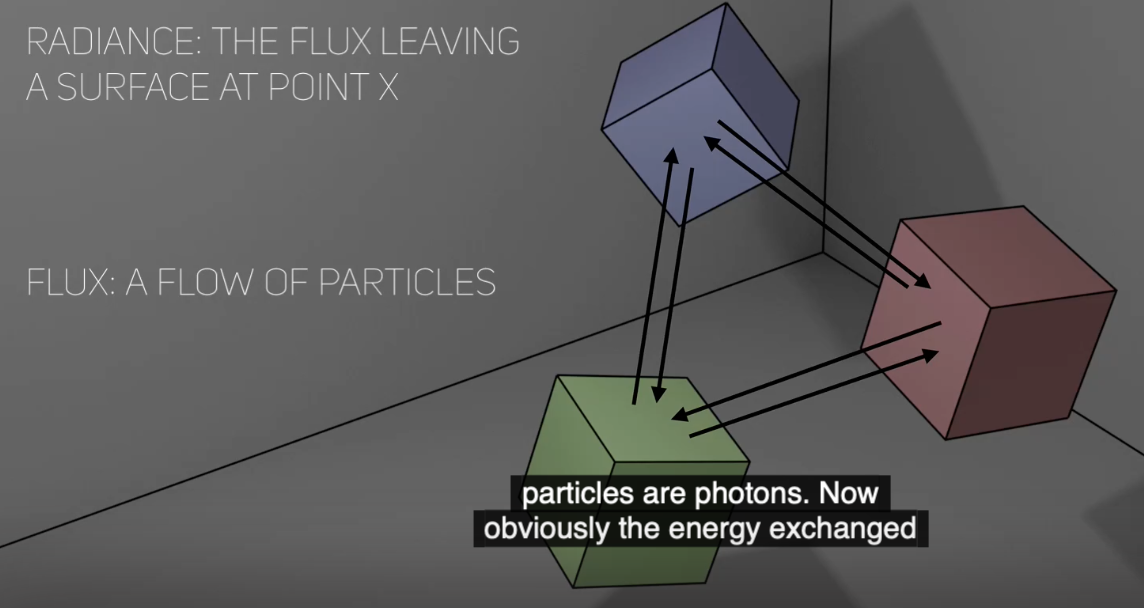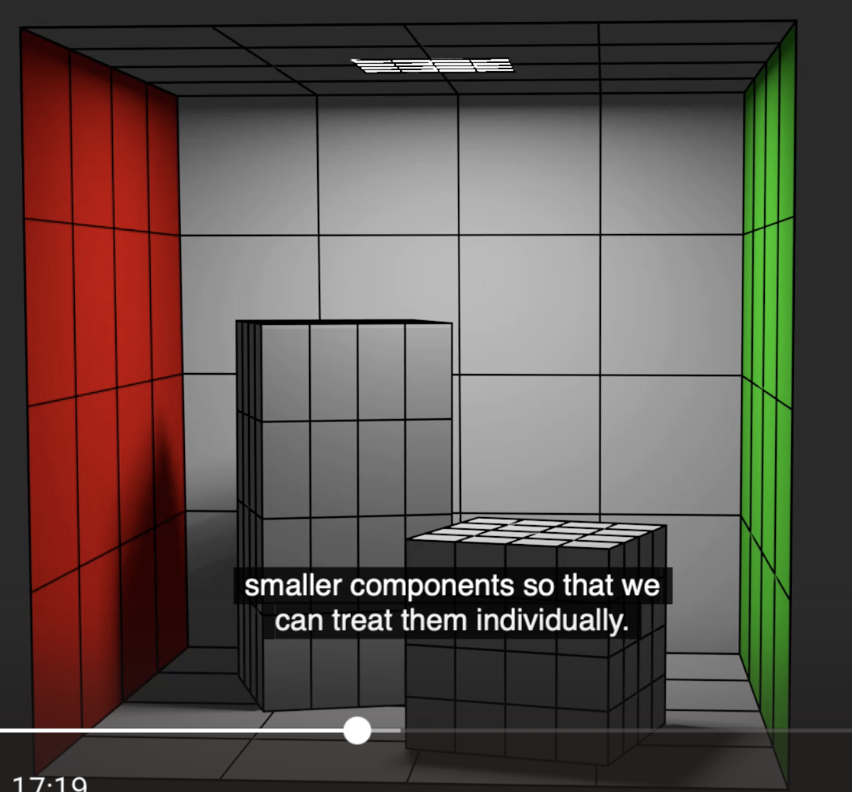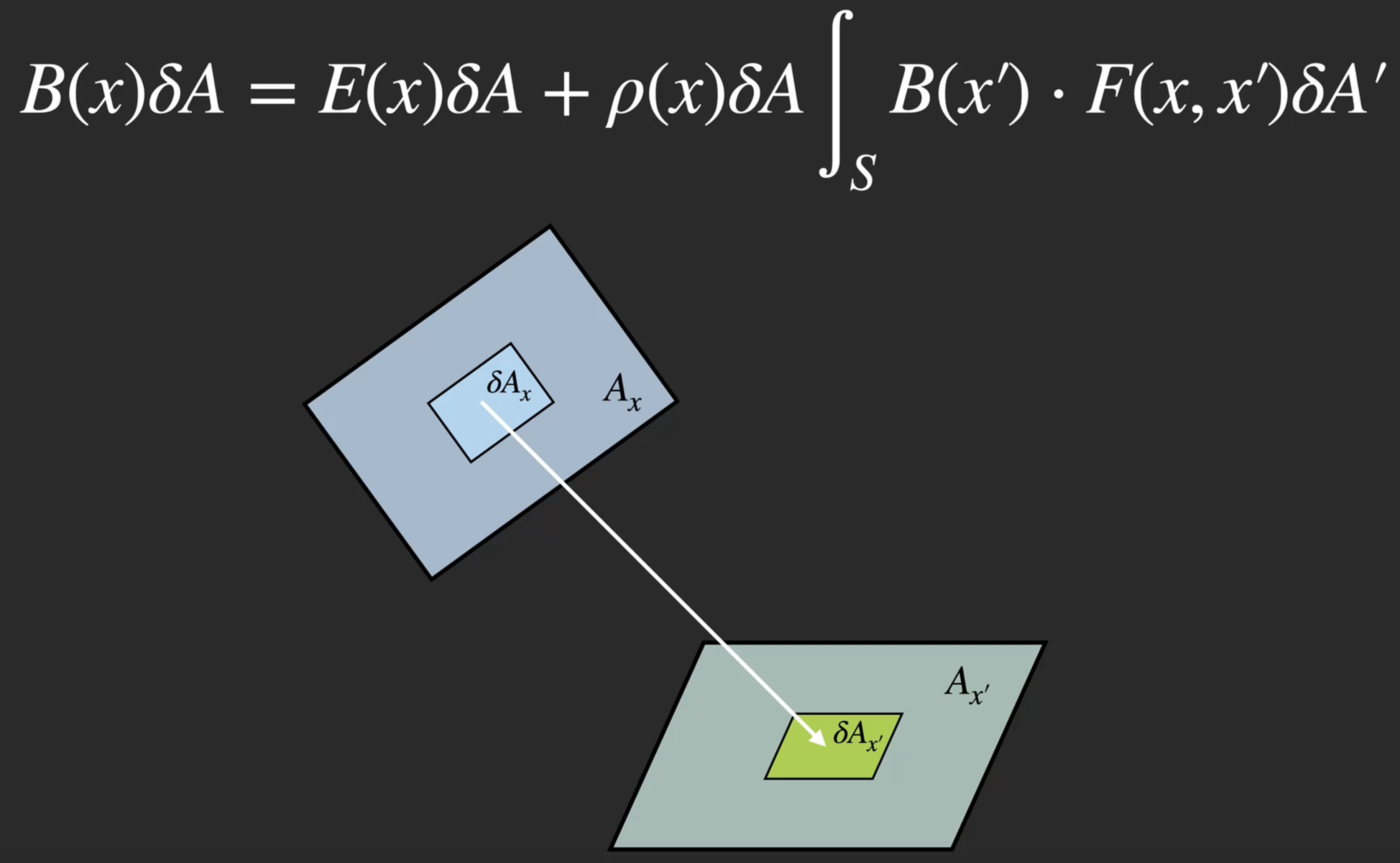$B(x)\delta A$

$E(x)\delta A$

$\rho(x)\delta A\int_{S} B(x') \cdot F(x, x') \delta A'$

$S$ 表示对变量 $x’$ 关于 整个场景 作积分, $F(x, x’)$ 表示这两个点之间的 Form Factor, 定义为: 当两个点 完全互相可见 时为 $1$, 反之为 $0$.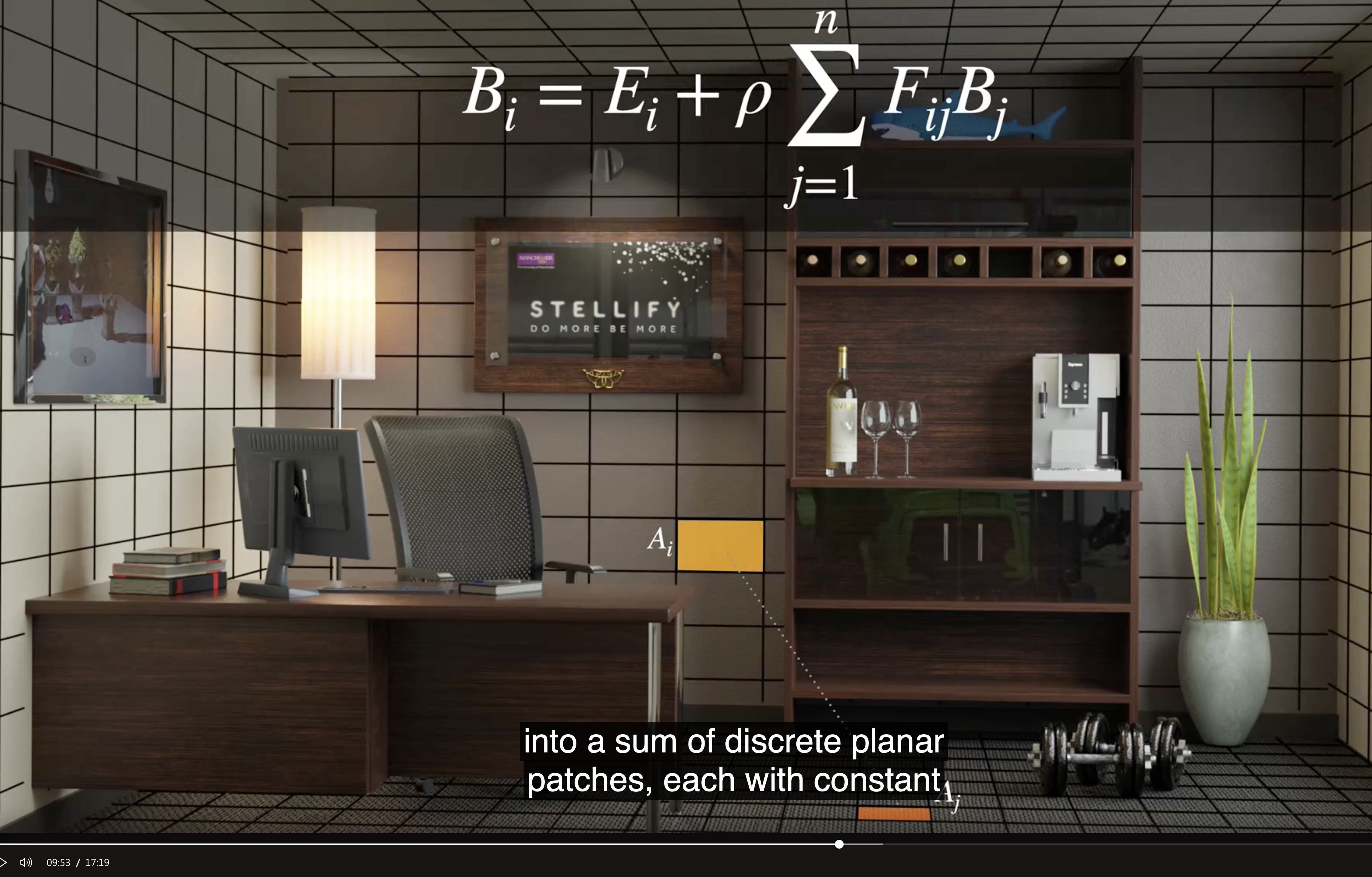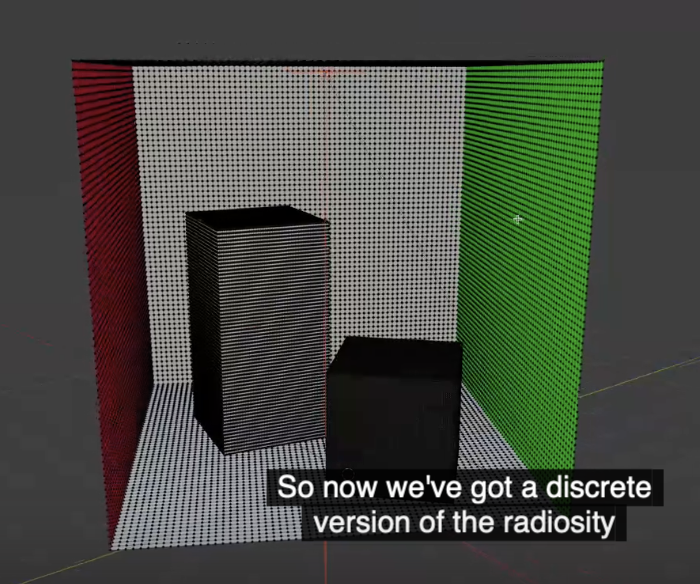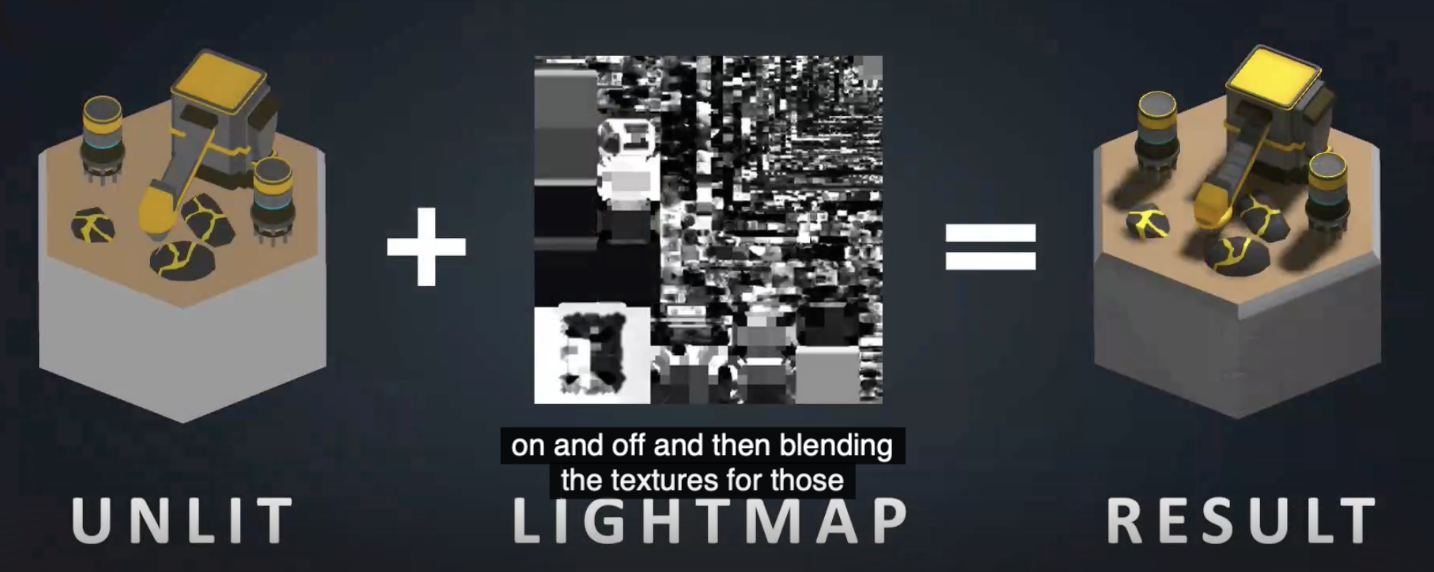viewpoint dependent: ray tracing, path tracing, direct volume rendering, proxy geometry (splatting)

viewpoint independent: radiosity, the rendering equation, texture baking, marching cubes